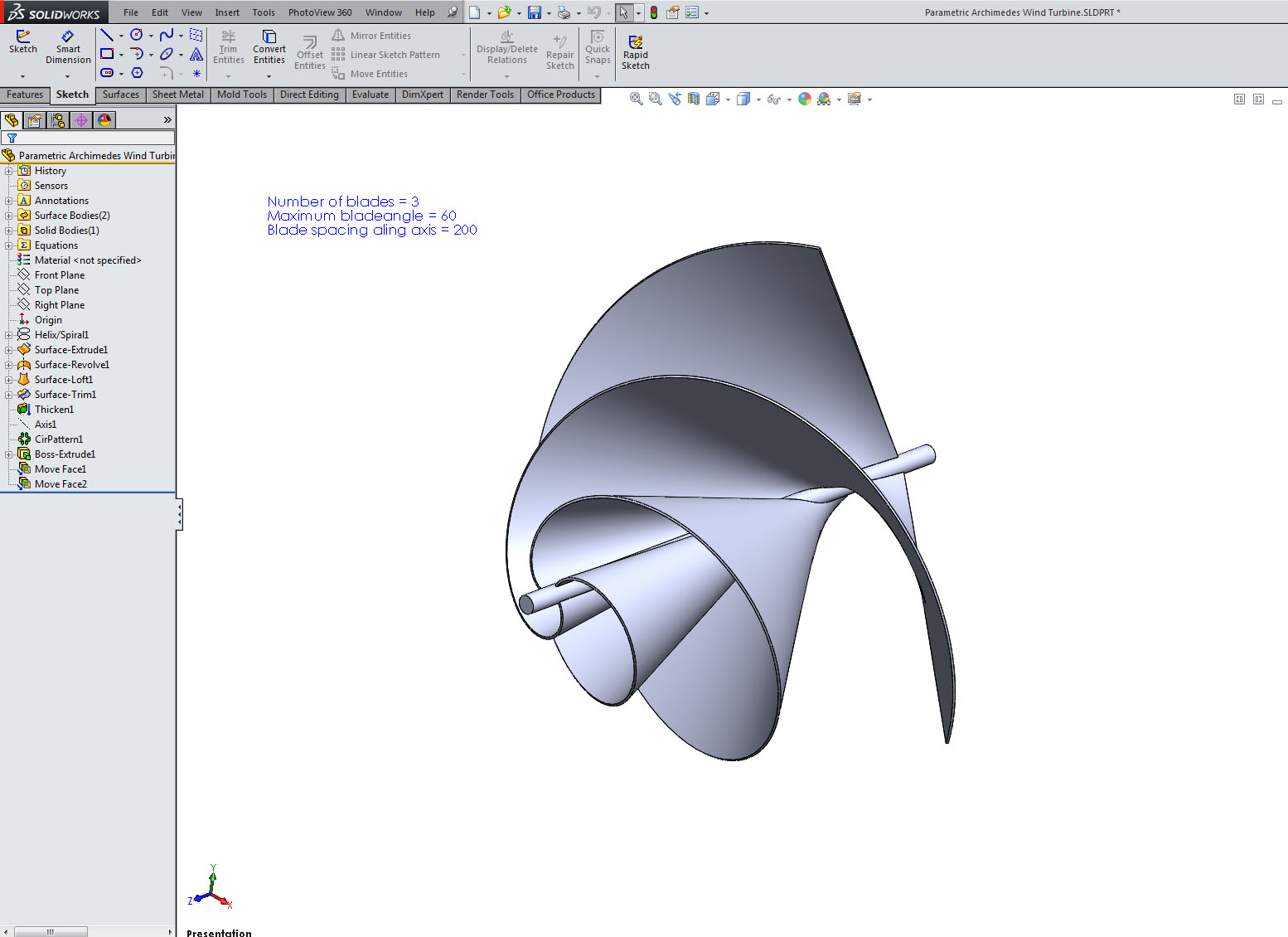# Question: How do I change the geometry of this parametric model?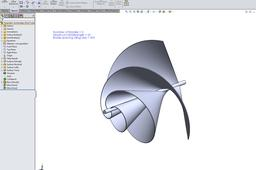The model is defined by four variables, that can be changed to generate new variations of the geometry.

1. ### Step 1:

Go to Insert->Tools->Equations to open the dialog box showing equations and variables.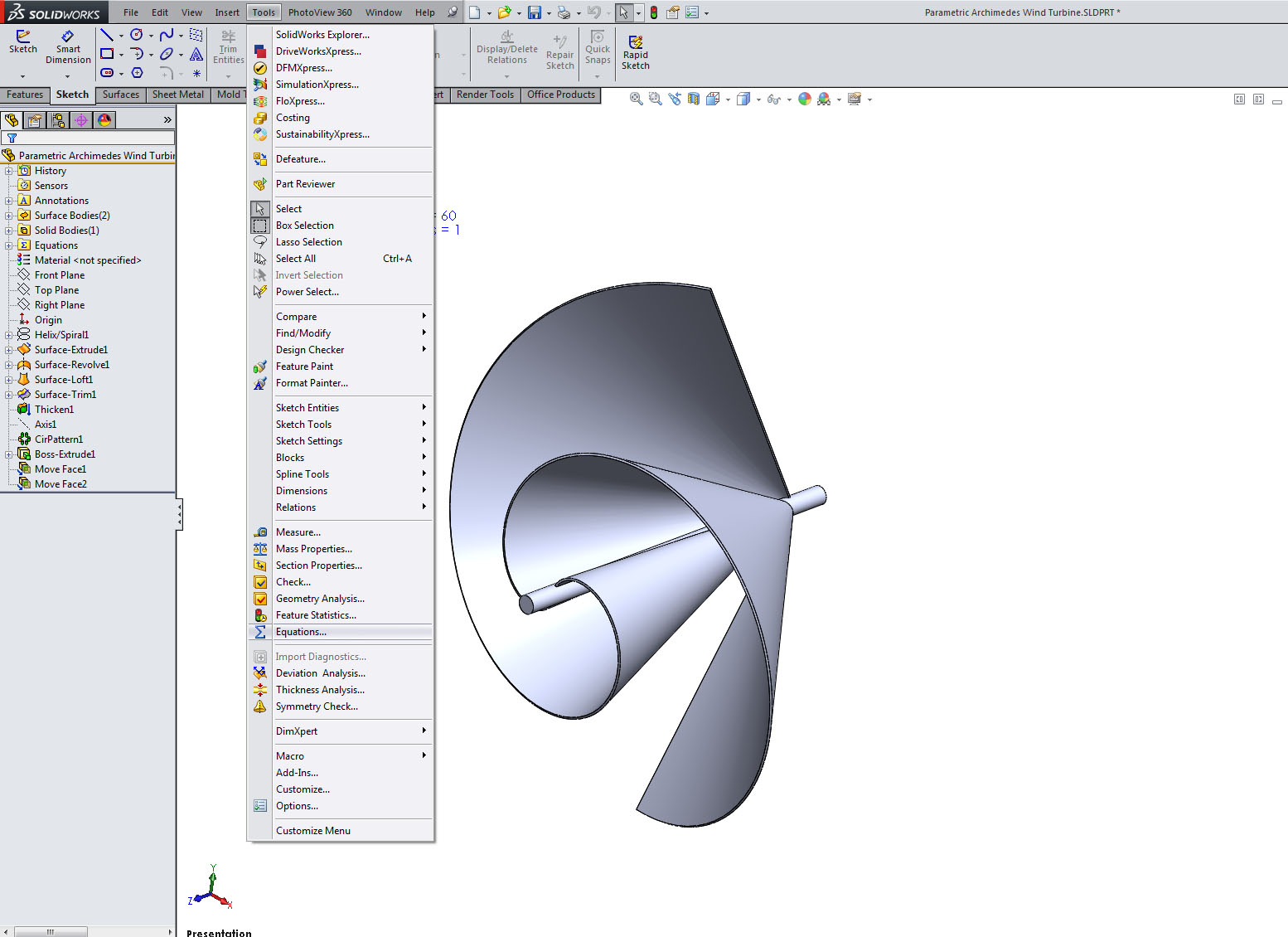2. ### Step 2:

Here you see the equations I have set-up in this particular model.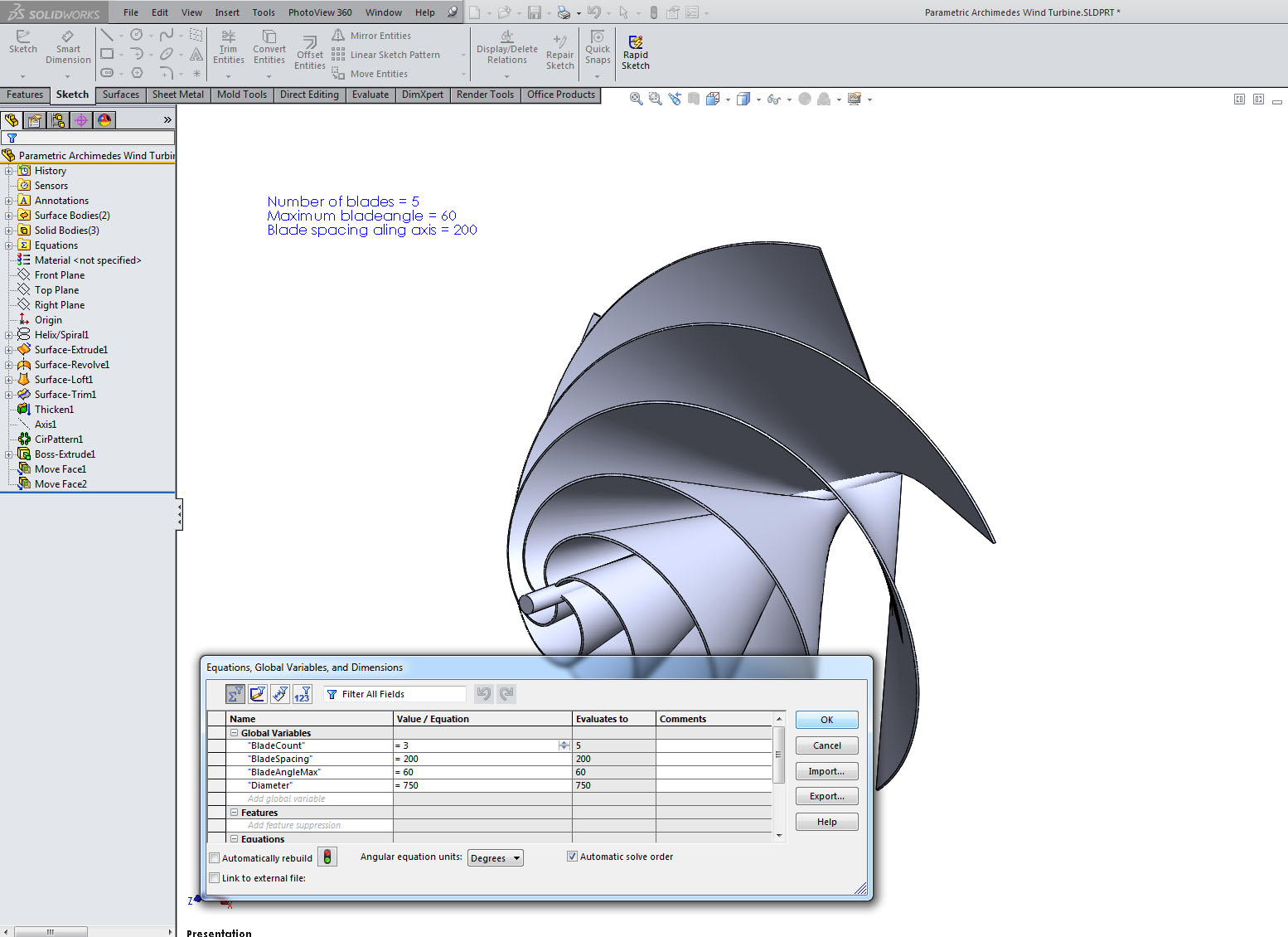3. ### Step 3:

Change any value and observe how the geometry changes when the model rebuilds.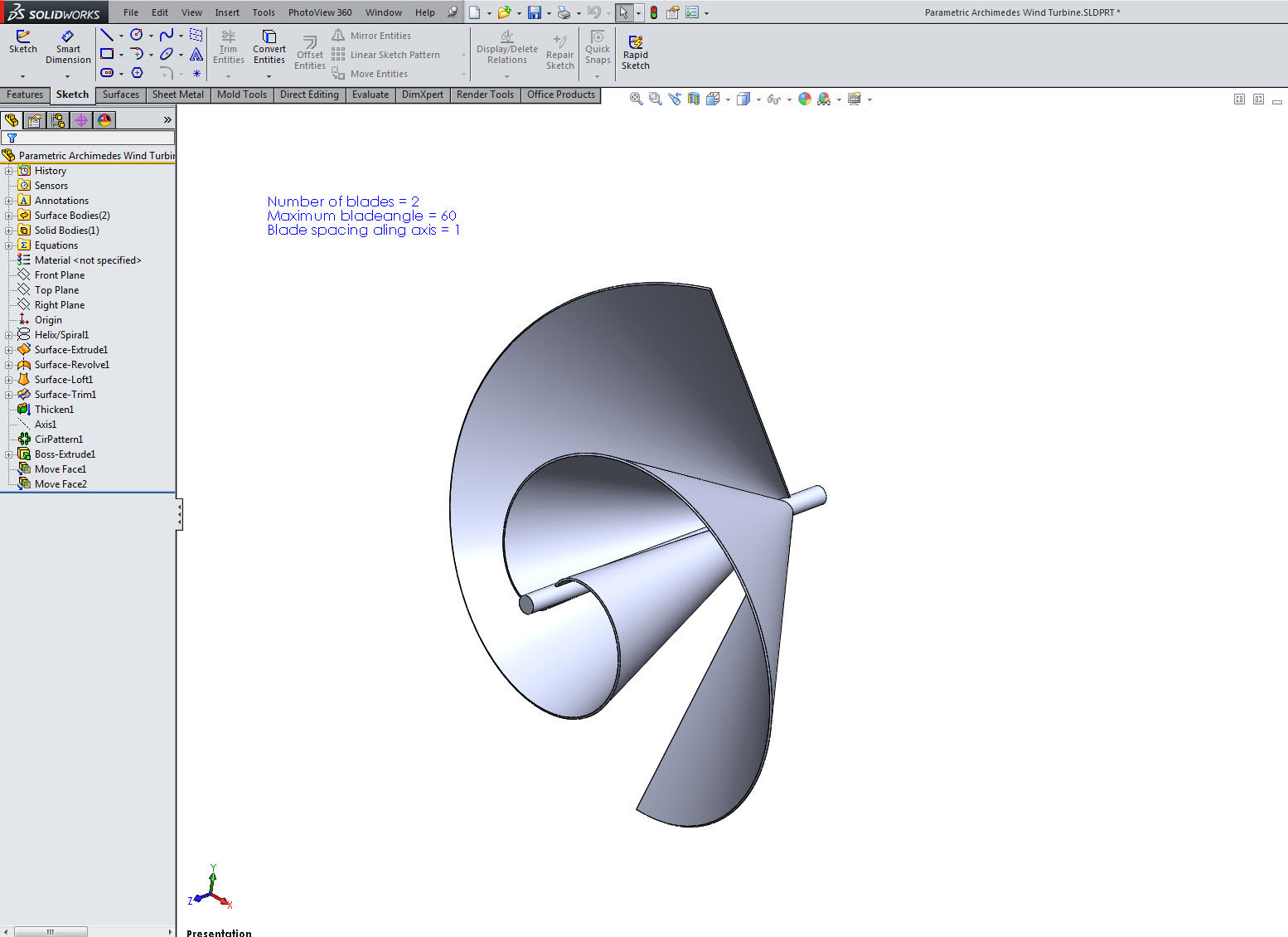4. ### Step 4: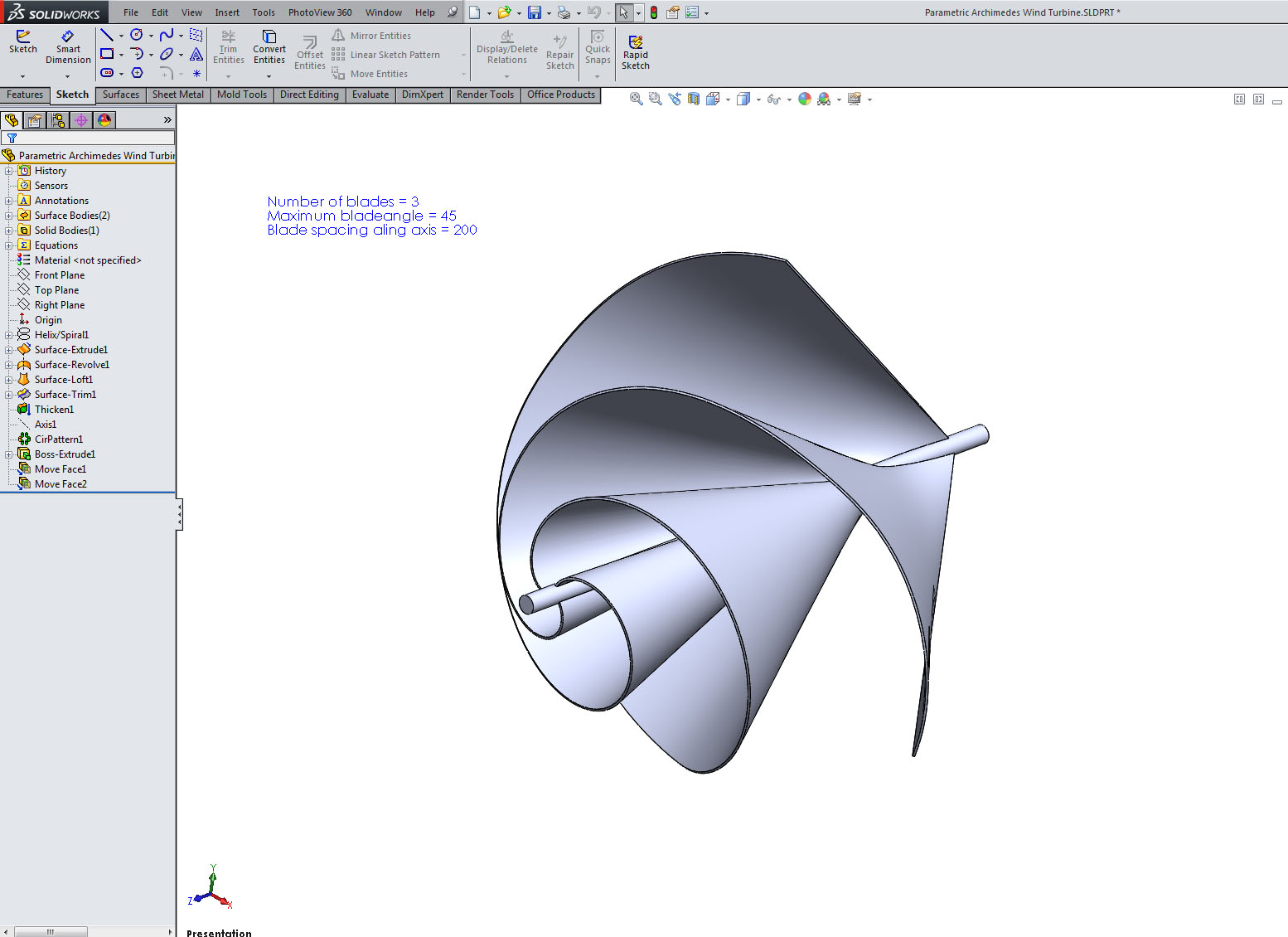5. ### Step 5:

You can create some pretty groovy variations...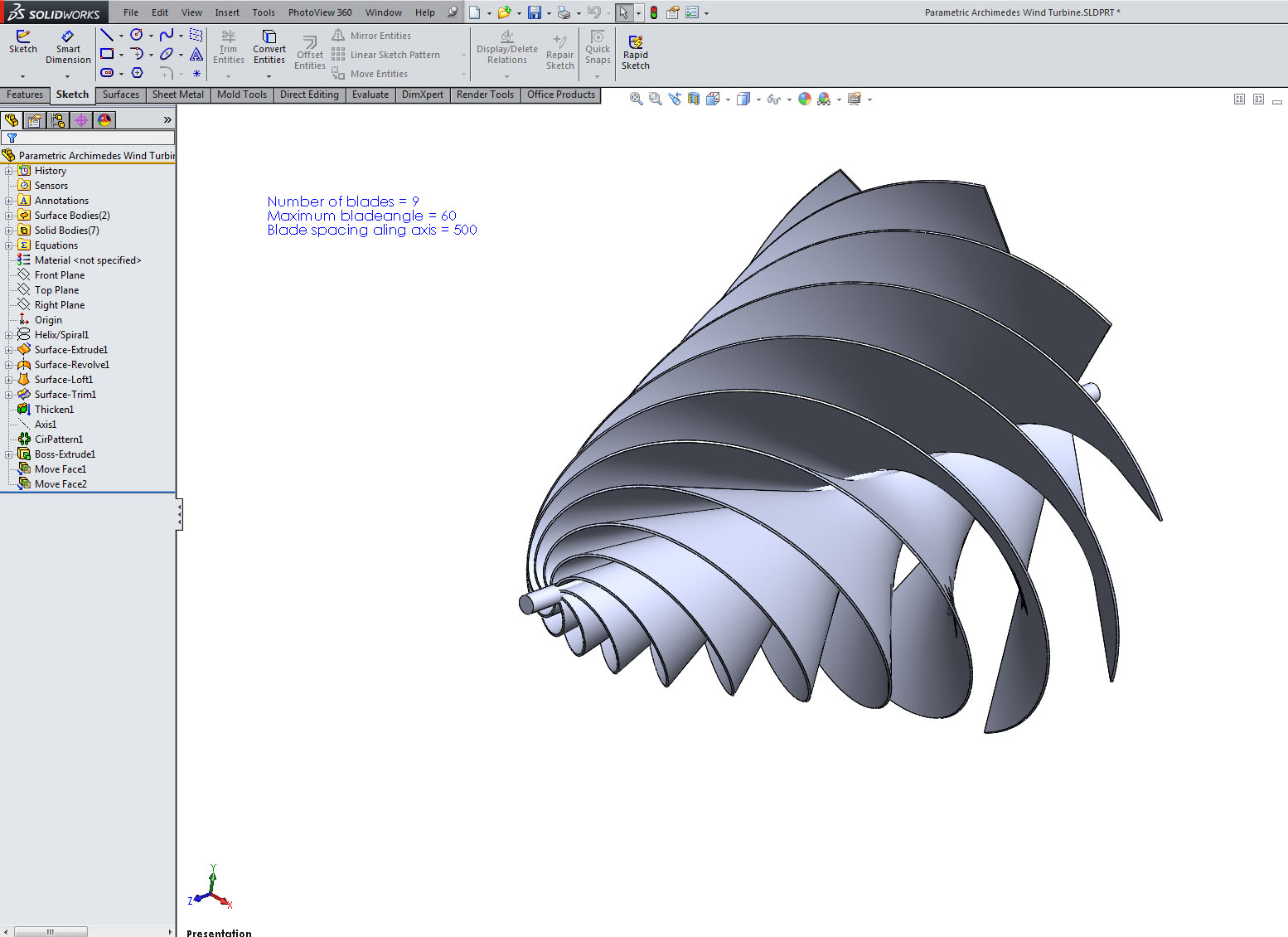6. ### Step 6:

...but be aware that some values will destroy the geometry. In that case re-open Equations and change back to the default values.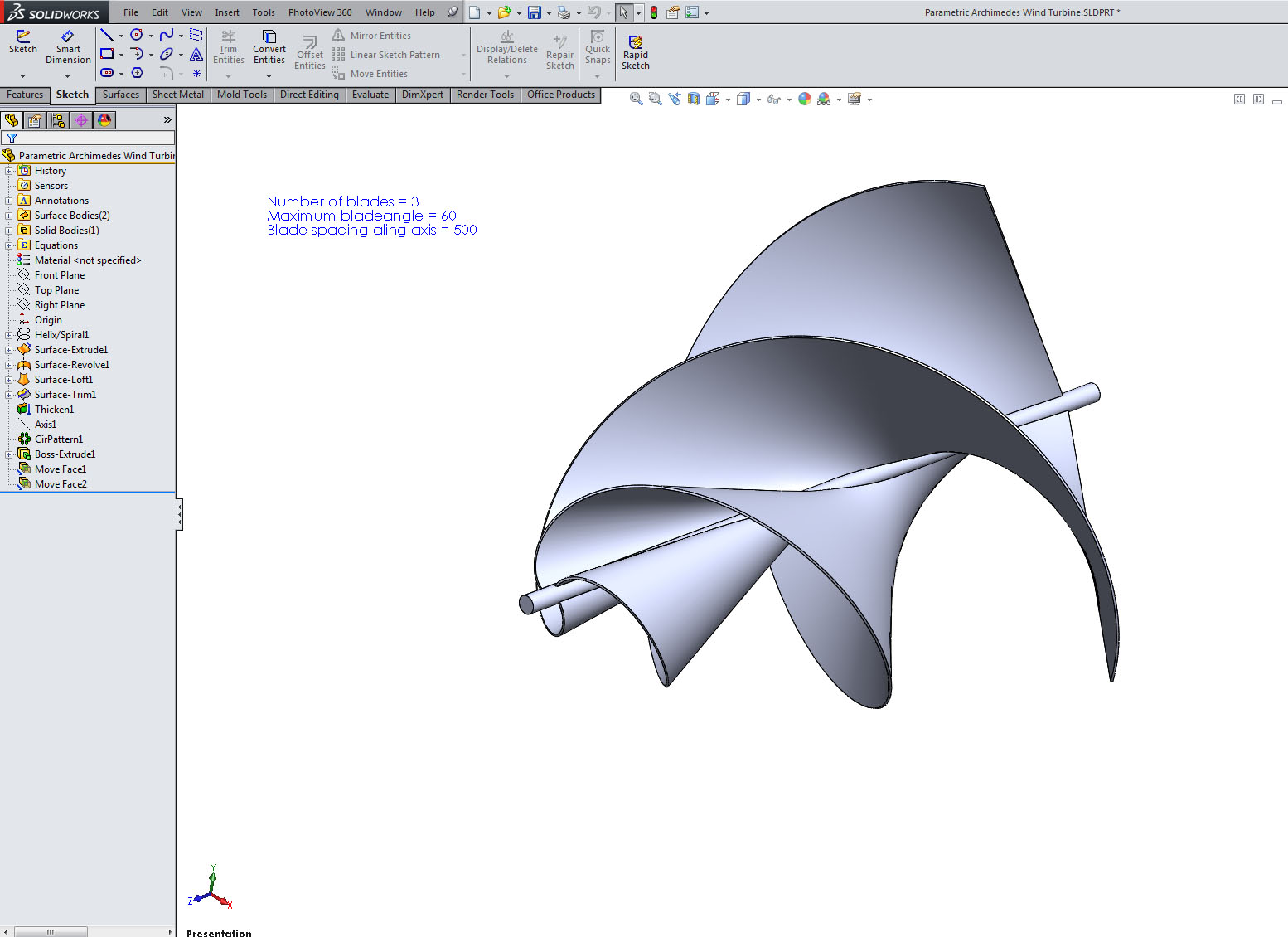7. ### Step 7:

The default values are 60 degree angle, 200 mm spacing, 3 blades and 750 mm diameter.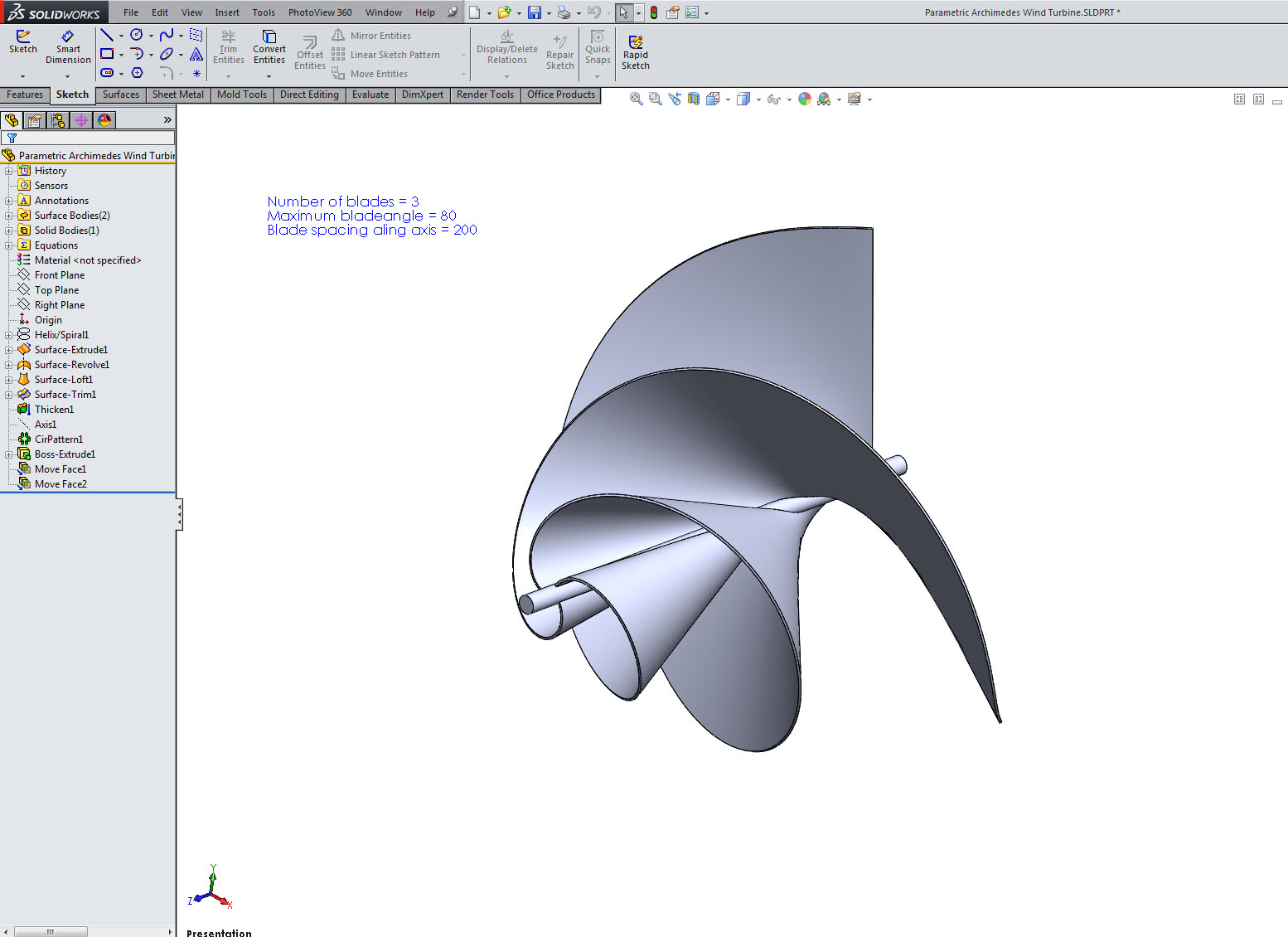8. ### Step 8:

This is the default model.